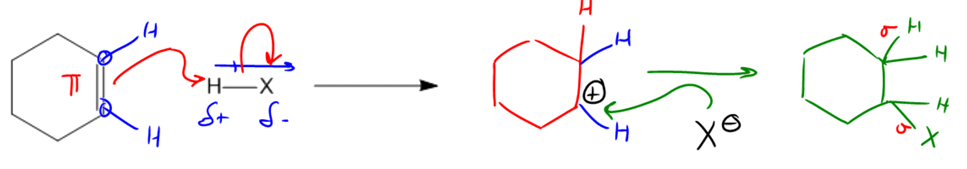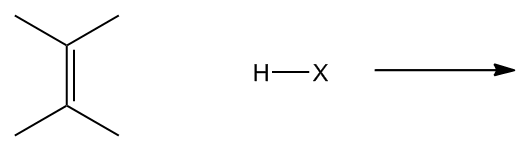Ch. 10 - Addition ReactionsWorksheetSee all chapters

See all sections
Sections
Markovnikov
Hydrohalogenation
Acid-Catalyzed Hydration
Oxymercuration
Hydroboration
Hydrogenation
Halogenation
Halohydrin
Carbene
Epoxidation
Epoxide Reactions
Dihydroxylation
Ozonolysis
Ozonolysis Full Mechanism
Oxidative Cleavage
Alkyne Oxidative Cleavage
Alkyne Hydrohalogenation
Alkyne Halogenation
Alkyne Hydration
Alkyne Hydroboration

Now we’re going to discuss a new type of organic mechanism called addition. There’s tons of these to keep track of, so let’s get right into it!

Concept #1: Features of Addition Mechanisms.

Transcript

Hey guys, so at the very beginning of this semester, in those first few lessons, I taught you guys that most of organic chemistry can just be summarized by the reaction of nucleophiles with electrophiles. And we've already seen a few examples of how negatively charged species are usually nucleophiles, but in this section what we're going to study is that double bonds can also be really good nucleophiles because they're good sources of electrons. And these reactions categorically are called addition reactions. So let's go ahead and get started.
So the general mechanism for an addition reaction is any reaction that's going to take one pi bond and it's going to break it and form two new sigma bonds. We call that addition because you're take basically taking one bond and you're making two later. So that makes sense. It's addition. You're basically adding to the number of bonds that the molecule has.
Now, this is actually going to be the inverse of a reaction that you may have already learned or are about to learn and that's called the elimination reaction. The elimination reaction is actually the exact opposite. All it means is that for an elimination you would take two sigma bonds and we'd make one pi. But in this case, what we're going to do is we're going to take that pi and we're going to make two sigma bonds.
Let's just look at the general mechanisms, see where the arrows would flow here. All the rules for mechanisms that I taught you in the past still apply to this mechanism. So where do you think the arrows would start from. Remember that we always want to start from the negatively charged species or the thing that's electron rich to the thing that's electron poor. So we're going to start from this double bond. The double bond is the source of electrons in this case. That is the nucleophile. So I'm going to draw an arrow coming from that double bond.
Now, maybe the more challenging part is figuring out where does this arrow go. In this case, I have an H and an X and I'm trying to find the electrophilic portion because I want to find what's the most positively charged thing on the map.
So how could we figure that out? Do you guys know? What we could use is dipoles. That's what we've been doing for a lot of molecules in this course. We've been saying where is the dipole going to pull. In this case, X stands for any of the halogens, so we know that X is always going to be more electronegative than the H.
So if I'm pulling electrons away from the H that means I would get a partial positive here and a partial negative here. Now we know what the electrophilic portion is. The electrophilic portion is going to be the H. So when I draw this mechanism, I'm going to draw it straight to that H.
Now can we just end there? Are we done? No, we're not done because remember hydrogen hates to have two bonds. And that new arrow represents a new bond that would be created. So if we make a bond, we have to break a bond. The bond that we would break is to the X. What this is going to create is a new molecule that looks like this. Where I now have basically a double bond and I'm going to have a new H on one side.
Now there's something that you have to realize here, these carbons right here already had how many hydrogens? They each had one. This had one hydrogen. This had one hydrogen. Is that cool? Because each of those carbons needed to have four bonds, obviously. Well, those hydrogens are still there. The only difference is that now I'm adding one more H. So let's go ahead and add one more H to one of those bonds. I'm just going to add it right here. That's the new H that's coming from my HX.
What about the bond at the bottom? Notice that I used to have a double bond on both of those carbons and now I don't anymore. What that means is that if I get an H on one side, I'm going to wind up getting a carbocation on the other. Now just remember, a carbocation is the name that we give to a carbon that's missing an electron. Instead of having four electrons, valence electrons, it only has three.
So now I've got this carbocation and I'm trying to figure out what to do with it. Is there anything it could react with? Yes. Well, check it out. I've actually got an X- hanging around. I've got a spare anion. So where do you think this next arrow is going to come from? The X. And I'm going to go ahead and attack the carbocation.
Do I have to draw another arrow now? Am I done? I actually am done because remember that when you make a bond, you only have to break a bond if you're violating the octet of that atom. By adding the X to the carbon, I'm actually going to complete the octet. So what that means is I don't have to break any more bonds. The mechanism is final drawn the way it is here.
What that means is that I'm going to get a product. And that product is going to be my addition product. What it's going to look like is that now I'm going to have, let's just say two H's here, and then I'm going to have an X here. Does that make sense so far? Cool.
So the reason we call this addition is because, check it out. I started off with one pi and at the end, I got two new sigmas out of it. Now, by the way, I drew all those H's just to be thorough, but you wouldn't need to draw all of them. In fact, you could just draw the product like this. Cyclohexane with an X because I know that the X is going to attach to one side or the other.
So just letting you know, that's the general mechanism. And it turns out that for this section we're going to be using the same mechanism over and over. We're going to use at least very similar mechanisms to this.

Addition reactions are ones in which 1 π-bond is broken and 2 new σ-bonds are formed.

They are the opposite of elimination reactions (if you haven’t learned about these yet don’t worry).Example #1: Provide the mechanism for the following addition reaction.